# RD Sharma Solutions Class 9 Maths Chapter 22 Tabular Representation Of Statistical Data Exercise 22.1

Class 9 Chapter 22 – Tabular Representation of Statistical Data Exercise 22.1 solutions are provided here. This exercise deals with the statistics data and frequency distribution. Frequency Distribution is a method to present raw data in the form which one can easily understand.

Frequency Distribution is of two types: Discrete Frequency Distribution and Continuous or Grouped Frequency Distribution.

Understand the concepts of the Cumulative frequency distribution by the solved examples that are easily explained in the RD Sharma solutions Class 9 Chapter 22. These solutions for class 9 textbook are outlined and solved by the experts at BYJU’S.

## Download PDF of RD Sharma Solutions for Class 9 Maths Chapter 22 Tabular Representation of Statistical Data Exercise 22.1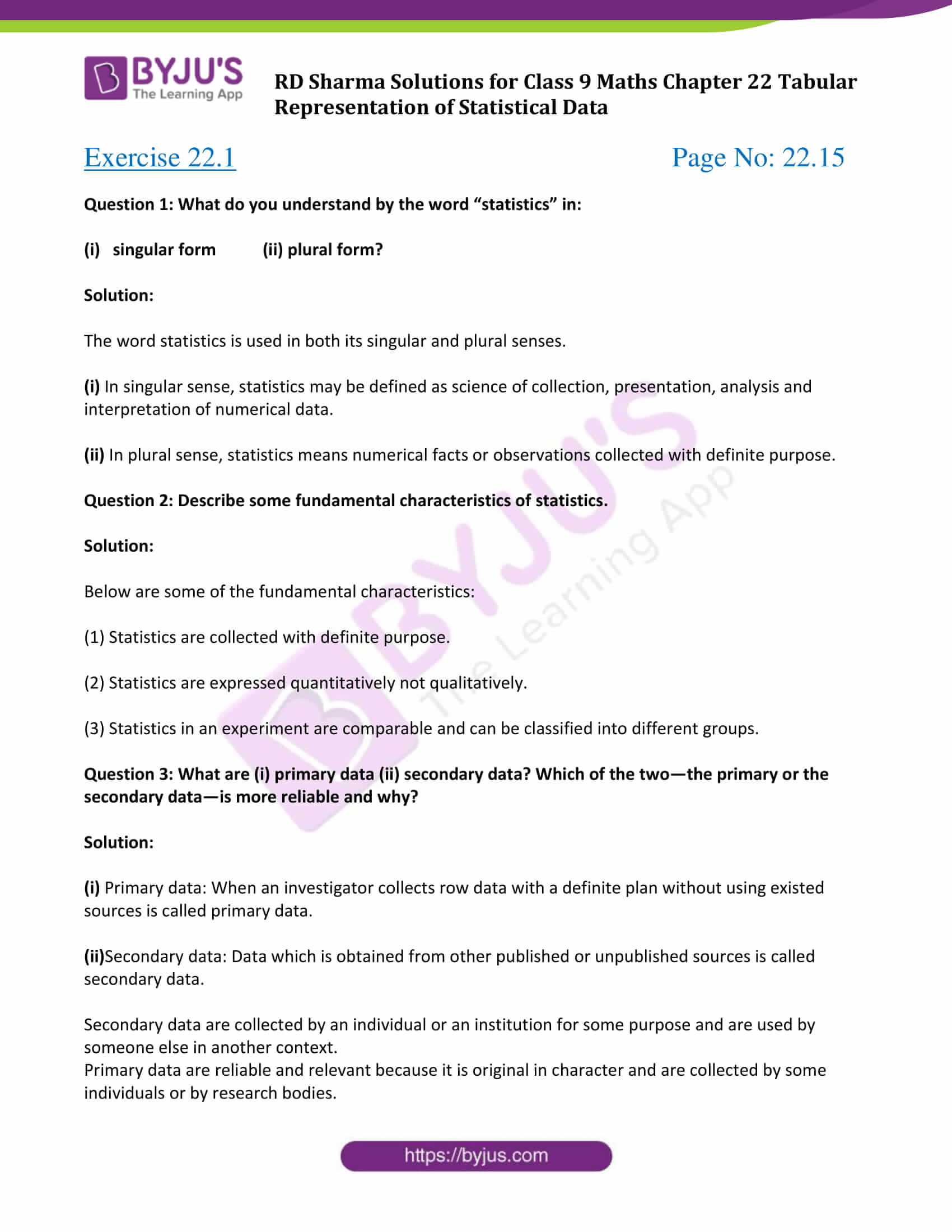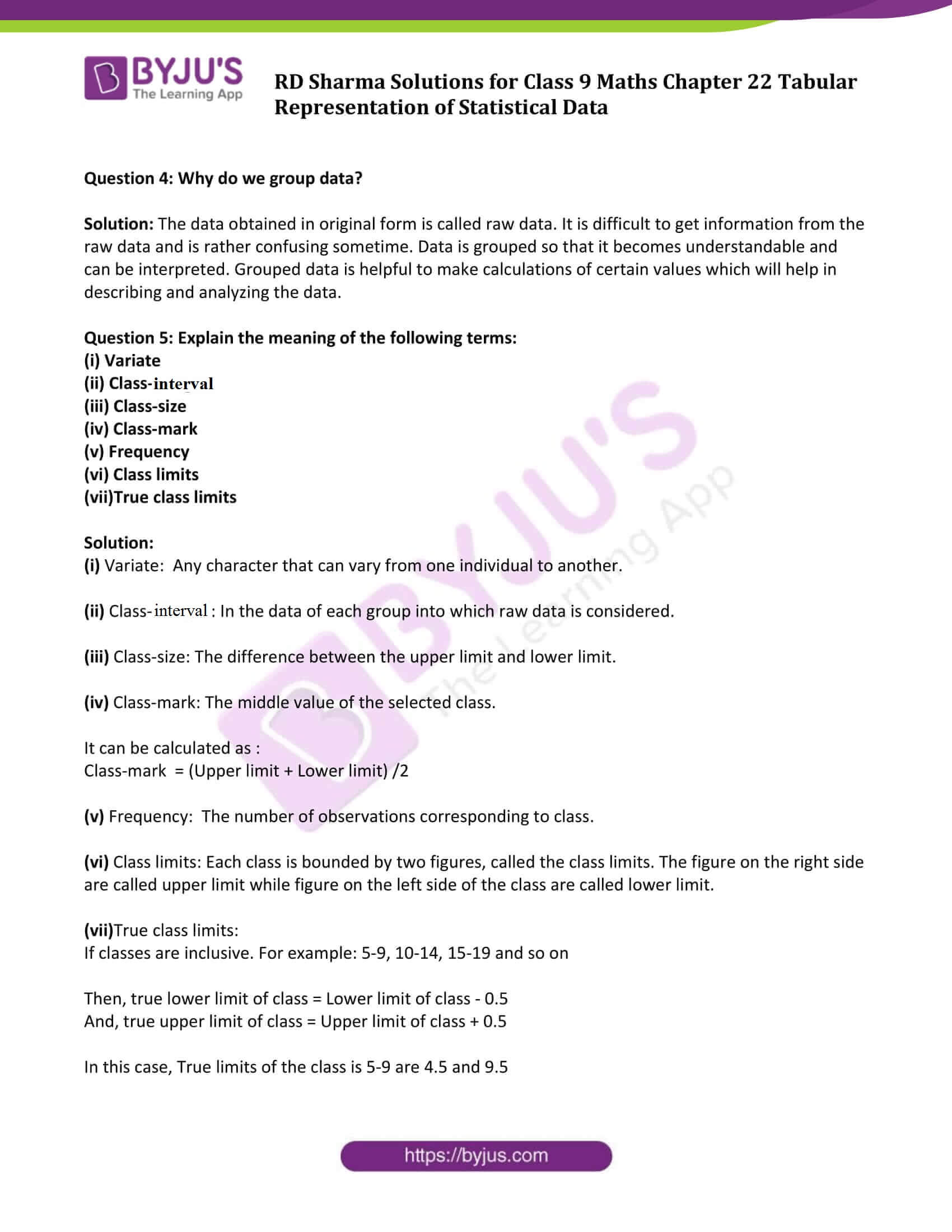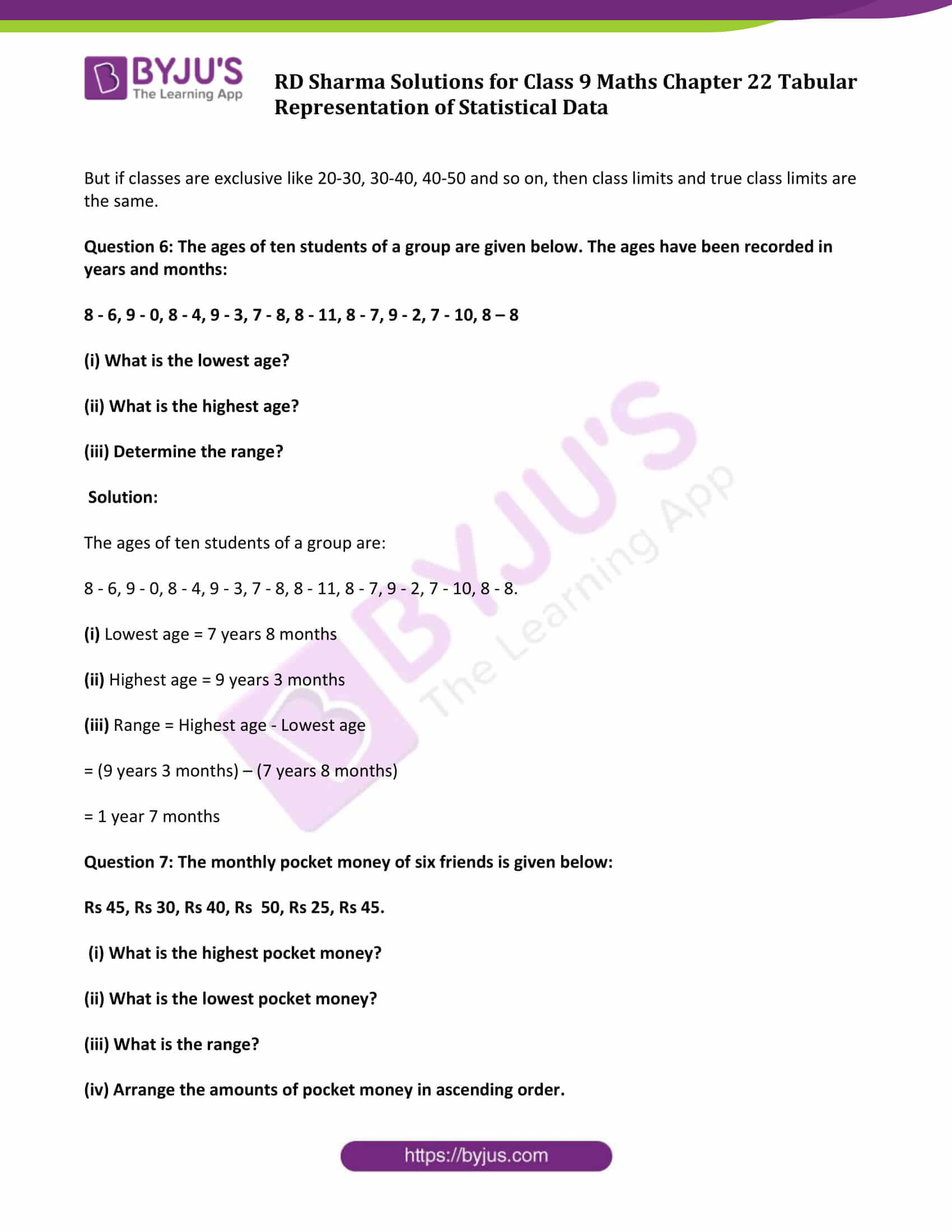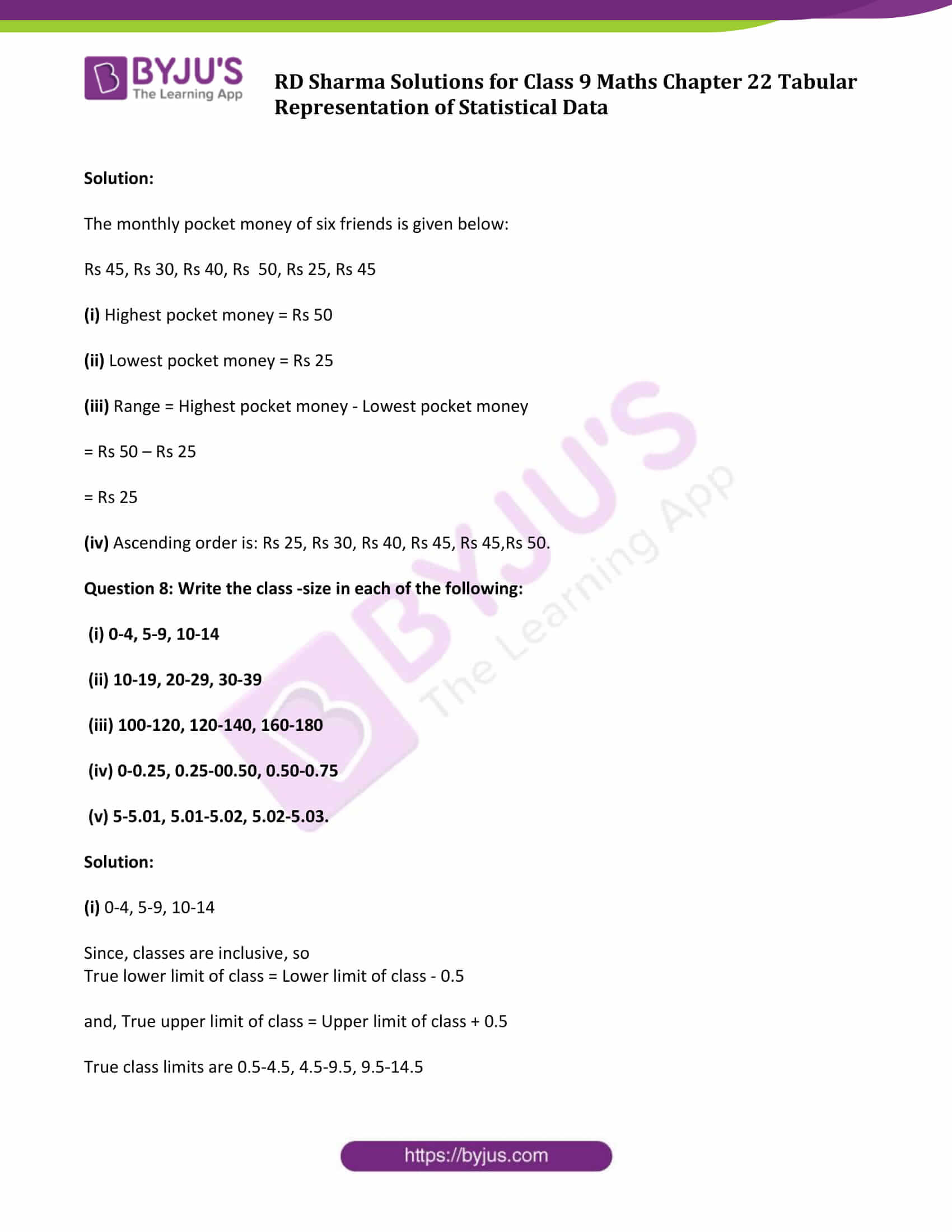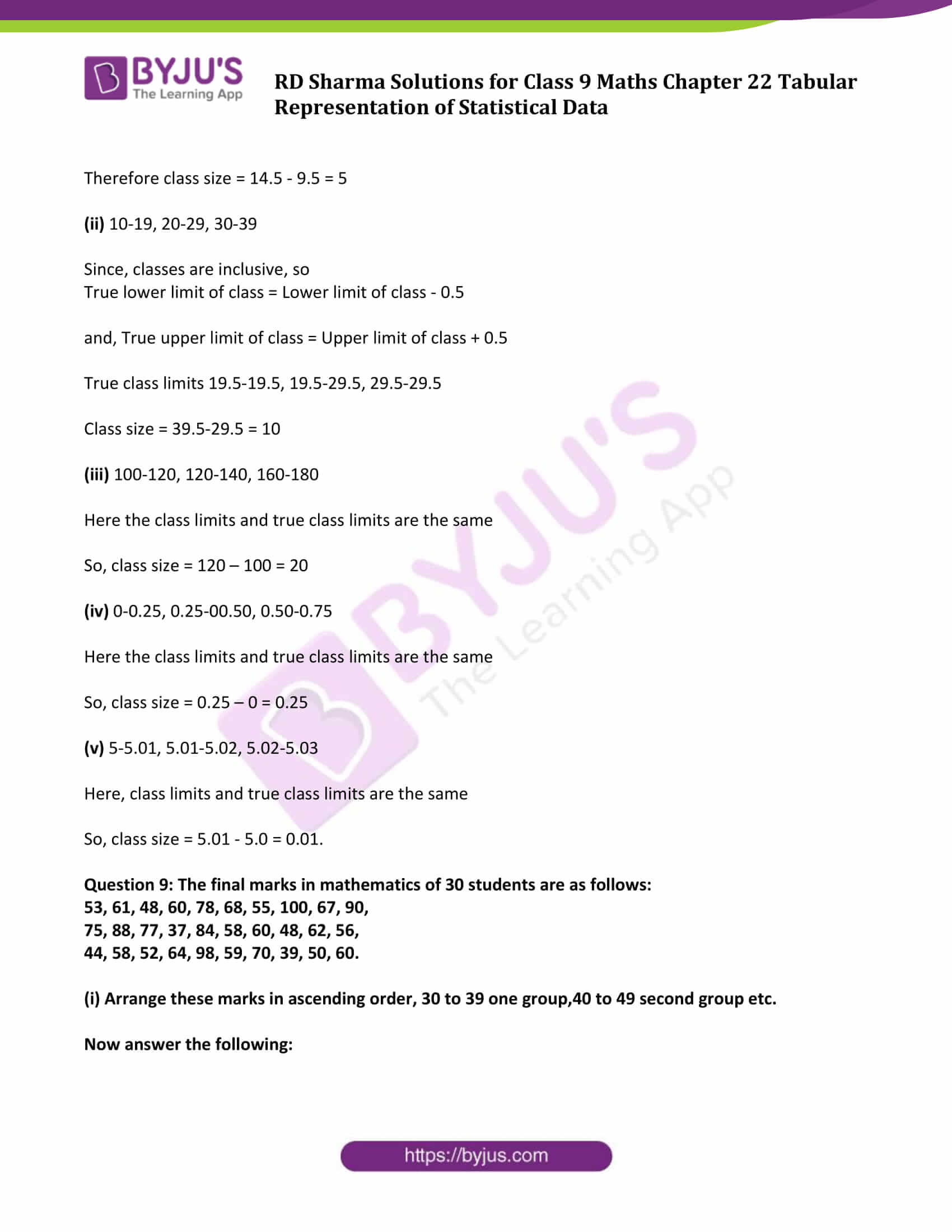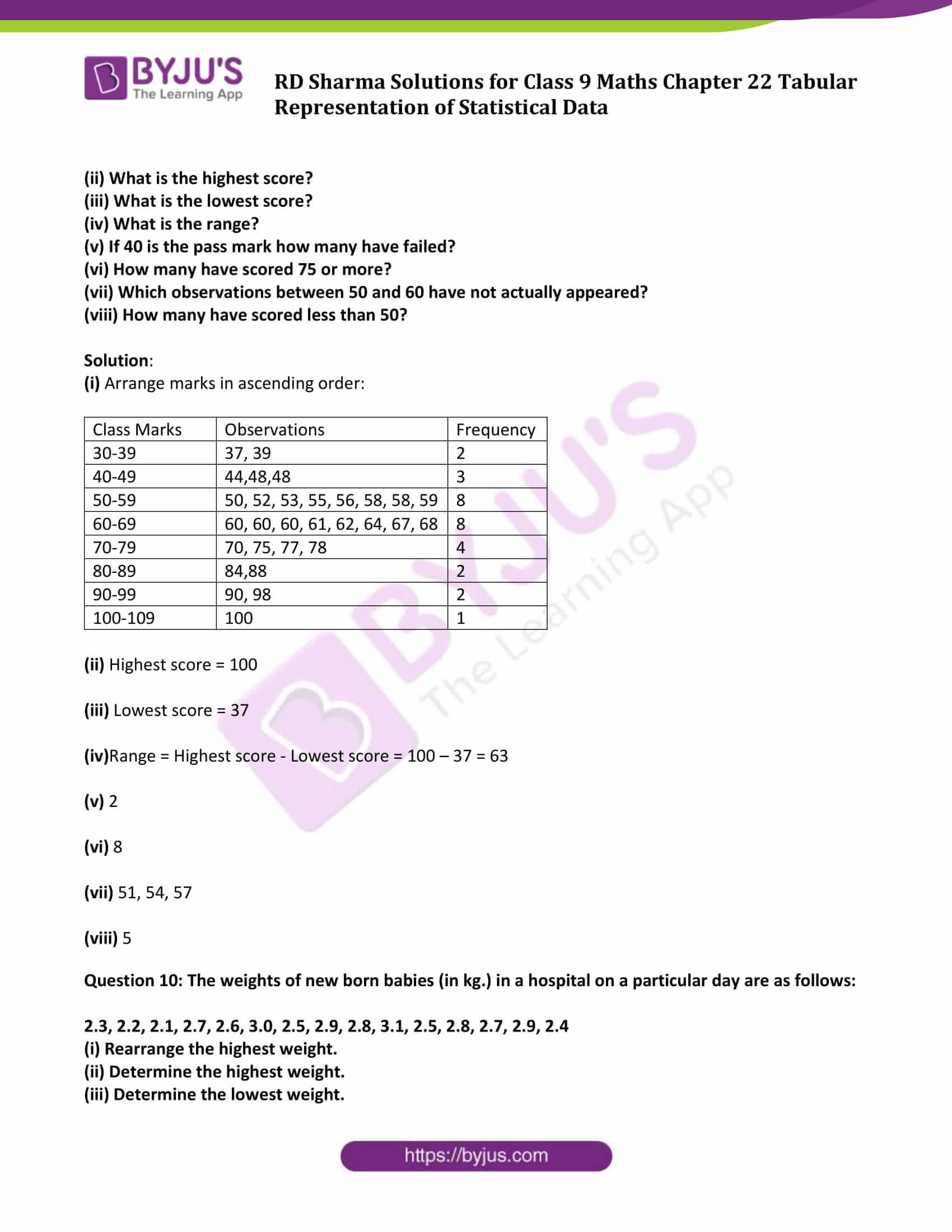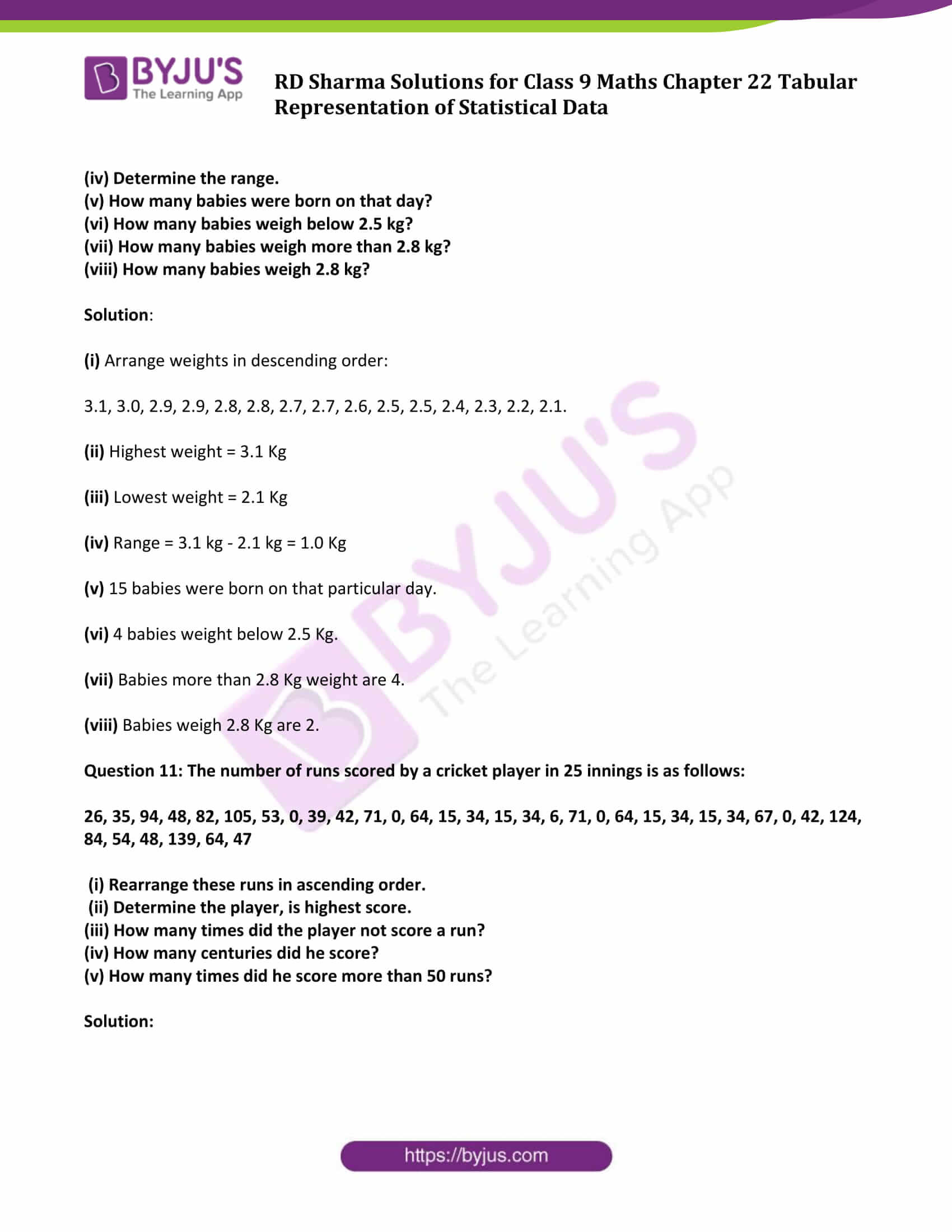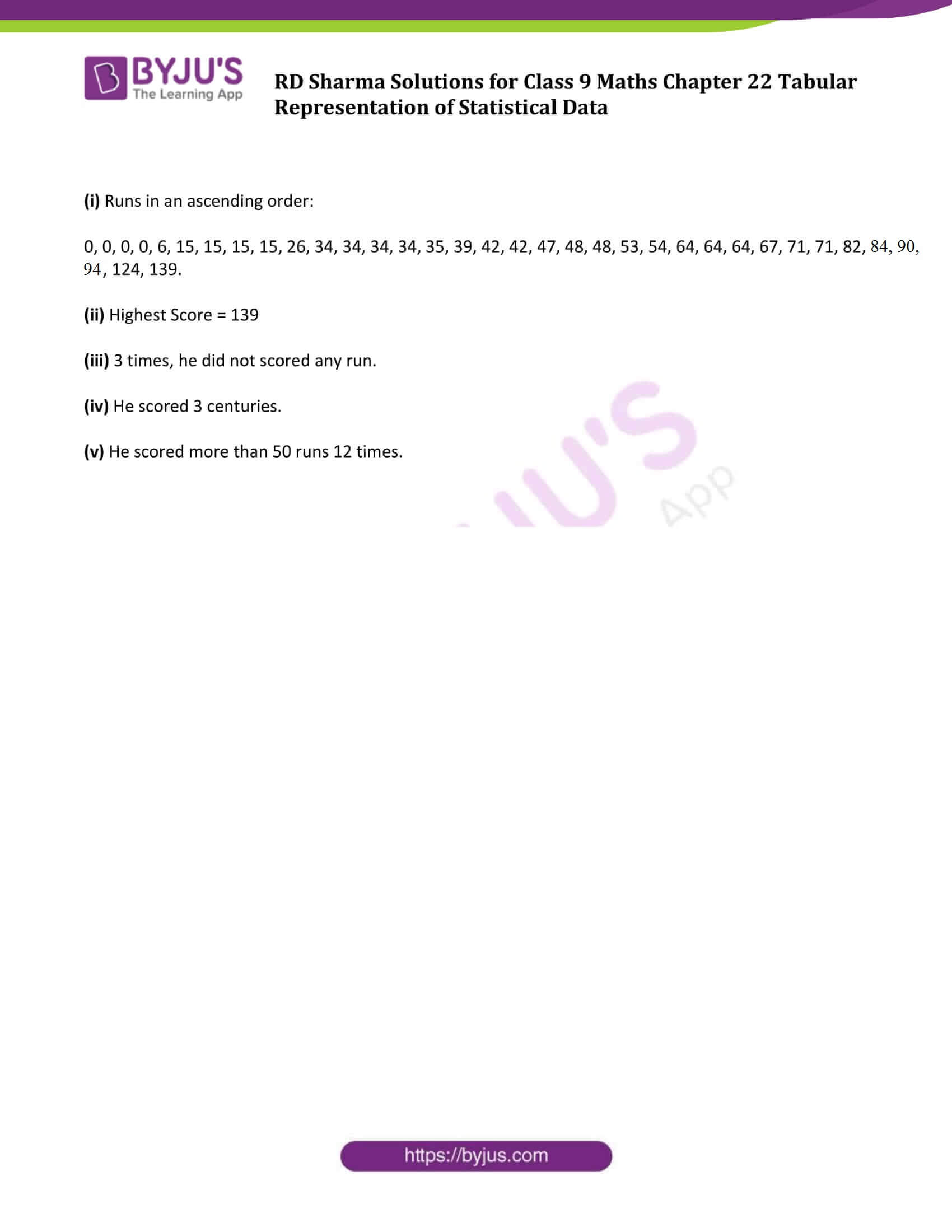### Access Answers to Maths RD Sharma Solutions for Class 9 Chapter 22 Tabular Representation of Statistical Data Exercise 22.1 Page number 22.15

Question 1: What do you understand by the word “statistics” in:

(i) singular form (ii) plural form?

Solution:

The word statistics is used in both its singular and plural senses.

(i) In singular sense, statistics may be defined as science of collection, presentation, analysis and interpretation of numerical data.

(ii) In plural sense, statistics means numerical facts or observations collected with definite purpose.

Question 2: Describe some fundamental characteristics of statistics.

Solution:

Below are some of the fundamental characteristics:

(1) Statistics are collected with definite purpose.

(2) Statistics are expressed quantitatively not qualitatively.

(3) Statistics in an experiment are comparable and can be classified into different groups.

Question 3: What are (i) primary data (ii) secondary data? Which of the two—the primary or the secondary data—is more reliable and why?

Solution:

(i) Primary data: When an investigator collects row data with a definite plan without using existed sources is called primary data.

(ii)Secondary data: Data which is obtained from other published or unpublished sources is called secondary data.

Secondary data are collected by an individual or an institution for some purpose and are used by someone else in another context.

Primary data are reliable and relevant because it is original in character and are collected by some individuals or by research bodies.

Question 4: Why do we group data?

Solution: The data obtained in original form is called raw data. It is difficult to get information from the raw data and is rather confusing sometime. Data is grouped so that it becomes understandable and can be interpreted. Grouped data is helpful to make calculations of certain values which will help in describing and analyzing the data.

Question 5: Explain the meaning of the following terms:

(i) Variate

(ii) Class-interval

(iii) Class-size

(iv) Class-mark

(v) Frequency

(vi) Class limits

(vii)True class limits

Solution:

(i) Variate: Any character that can vary from one individual to another.

(ii) Class-interval: In the data of each group into which raw data is considered.

(iii) Class-size: The difference between the upper limit and lower limit.

(iv) Class-mark: The middle value of the selected class.

It can be calculated as :

Class-mark = (Upper limit + Lower limit) /2

(v) Frequency: The number of observations corresponding to class.

(vi) Class limits: Each class is bounded by two figures, called the class limits. The figure on the right side are called upper limit while figure on the left side of the class are called lower limit.

(vii)True class limits:

If classes are inclusive. For example: 5-9, 10-14, 15-19 and so on

Then, true lower limit of class = Lower limit of class – 0.5

And, true upper limit of class = Upper limit of class + 0.5

In this case, True limits of the class is 5-9 are 4.5 and 9.5

But if classes are exclusive like 20-30, 30-40, 40-50 and so on, then class limits and true class limits are the same.

Question 6: The ages of ten students of a group are given below. The ages have been recorded in years and months:

8 – 6, 9 – 0, 8 – 4, 9 – 3, 7 – 8, 8 – 11, 8 – 7, 9 – 2, 7 – 10, 8 – 8

(i) What is the lowest age?

(ii) What is the highest age?

(iii) Determine the range?

Solution:

The ages of ten students of a group are:

8 – 6, 9 – 0, 8 – 4, 9 – 3, 7 – 8, 8 – 11, 8 – 7, 9 – 2, 7 – 10, 8 – 8.

(i) Lowest age = 7 years 8 months

(ii) Highest age = 9 years 3 months

(iii) Range = Highest age – Lowest age

= (9 years 3 months) – (7 years 8 months)

= 1 year 7 months

Question 7: The monthly pocket money of six friends is given below:

Rs 45, Rs 30, Rs 40, Rs 50, Rs 25, Rs 45.

(i) What is the highest pocket money?

(ii) What is the lowest pocket money?

(iii) What is the range?

(iv) Arrange the amounts of pocket money in ascending order.

Solution:

The monthly pocket money of six friends is given below:

Rs 45, Rs 30, Rs 40, Rs 50, Rs 25, Rs 45

(i) Highest pocket money = Rs 50

(ii) Lowest pocket money = Rs 25

(iii) Range = Highest pocket money – Lowest pocket money

= Rs 50 – Rs 25

= Rs 25

(iv) Ascending order is: Rs 25, Rs 30, Rs 40, Rs 45, Rs 45,Rs 50.

Question 8: Write the class -size in each of the following:

(i) 0-4, 5-9, 10-14

(ii) 10-19, 20-29, 30-39

(iii) 100-120, 120-140, 160-180

(iv) 0-0.25, 0.25-00.50, 0.50-0.75

(v) 5-5.01, 5.01-5.02, 5.02-5.03.

Solution:

(i) 0-4, 5-9, 10-14

Since, classes are inclusive, so

True lower limit of class = Lower limit of class – 0.5

and, True upper limit of class = Upper limit of class + 0.5

True class limits are 0.5-4.5, 4.5-9.5, 9.5-14.5

Therefore class size = 14.5 – 9.5 = 5

(ii) 10-19, 20-29, 30-39

Since, classes are inclusive, so

True lower limit of class = Lower limit of class – 0.5

and, True upper limit of class = Upper limit of class + 0.5

True class limits 19.5-19.5, 19.5-29.5, 29.5-29.5

Class size = 39.5-29.5 = 10

(iii) 100-120, 120-140, 160-180

Here the class limits and true class limits are the same

So, class size = 120 – 100 = 20

(iv) 0-0.25, 0.25-00.50, 0.50-0.75

Here the class limits and true class limits are the same

So, class size = 0.25 – 0 = 0.25

(v) 5-5.01, 5.01-5.02, 5.02-5.03

Here, class limits and true class limits are the same

So, class size = 5.01 – 5.0 = 0.01.

Question 9: The final marks in mathematics of 30 students are as follows:

53, 61, 48, 60, 78, 68, 55, 100, 67, 90,

75, 88, 77, 37, 84, 58, 60, 48, 62, 56,

44, 58, 52, 64, 98, 59, 70, 39, 50, 60.

(i) Arrange these marks in ascending order, 30 to 39 one group,40 to 49 second group etc.

(ii) What is the highest score?

(iii) What is the lowest score?

(iv) What is the range?

(v) If 40 is the pass mark how many have failed?

(vi) How many have scored 75 or more?

(vii) Which observations between 50 and 60 have not actually appeared?

(viii) How many have scored less than 50?

Solution:

(i) Arrange marks in ascending order:

 Class Marks Observations Frequency 30-39 37, 39 2 40-49 44,48,48 3 50-59 50, 52, 53, 55, 56, 58, 58, 59 8 60-69 60, 60, 60, 61, 62, 64, 67, 68 8 70-79 70, 75, 77, 78 4 80-89 84,88 2 90-99 90, 98 2 100-109 100 1

(ii) Highest score = 100

(iii) Lowest score = 37

(iv)Range = Highest score – Lowest score = 100 – 37 = 63

(v) 2

(vi) 8

(vii) 51, 54, 57

(viii) 5

Question 10: The weights of new born babies (in kg.) in a hospital on a particular day are as follows:

2.3, 2.2, 2.1, 2.7, 2.6, 3.0, 2.5, 2.9, 2.8, 3.1, 2.5, 2.8, 2.7, 2.9, 2.4

(i) Rearrange the highest weight.

(ii) Determine the highest weight.

(iii) Determine the lowest weight.

(iv) Determine the range.

(v) How many babies were born on that day?

(vi) How many babies weigh below 2.5 kg?

(vii) How many babies weigh more than 2.8 kg?

(viii) How many babies weigh 2.8 kg?

Solution:

(i) Arrange weights in descending order:

3.1, 3.0, 2.9, 2.9, 2.8, 2.8, 2.7, 2.7, 2.6, 2.5, 2.5, 2.4, 2.3, 2.2, 2.1.

(ii) Highest weight = 3.1 Kg

(iii) Lowest weight = 2.1 Kg

(iv) Range = 3.1 kg – 2.1 kg = 1.0 Kg

(v) 15 babies were born on that particular day.

(vi) 4 babies weight below 2.5 Kg.

(vii) Babies more than 2.8 Kg weight are 4.

(viii) Babies weigh 2.8 Kg are 2.

Question 11: The number of runs scored by a cricket player in 25 innings is as follows:

26, 35, 94, 48, 82, 105, 53, 0, 39, 42, 71, 0, 64, 15, 34, 15, 34, 6, 71, 0, 64, 15, 34, 15, 34, 67, 0, 42, 124, 84, 54, 48, 139, 64, 47

(i) Rearrange these runs in ascending order.

(ii) Determine the player, is highest score.

(iii) How many times did the player not score a run?

(iv) How many centuries did he score?

(v) How many times did he score more than 50 runs?

Solution:

(i) Runs in an ascending order:

0, 0, 0, 0, 6, 15, 15, 15, 15, 26, 34, 34, 34, 34, 35, 39, 42, 42, 47, 48, 48, 53, 54, 64, 64, 64, 67, 71, 71, 82, 84, 90, 94, 124, 139.

(ii) Highest Score = 139

(iii) 3 times, he did not scored any run.

(iv) He scored 3 centuries.

(v) He scored more than 50 runs 12 times.

## RD Sharma Solutions for Class 9 Maths Chapter 22 Tabular Representation of Statistical Data Exercise 22.1

RD Sharma Solutions Class 9 Maths Chapter 22 Tabular Representation of Statistical Data Exercise 22.1 is based on the following topics:

• Statistics data

-Primary data

-Secondary data

• Presentation of data
• Frequency distribution

-Discrete frequency distribution

– Continuous or grouped frequency distribution

• Construction of a Discrete frequency distribution
• Construction of a continuous or grouped frequency distribution